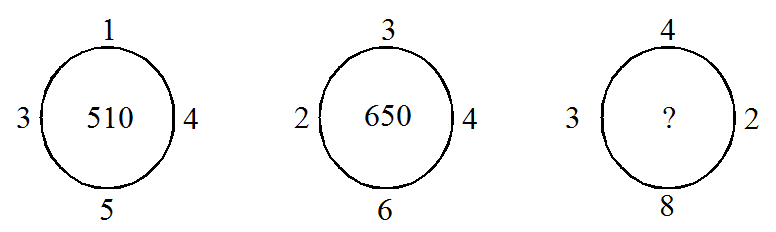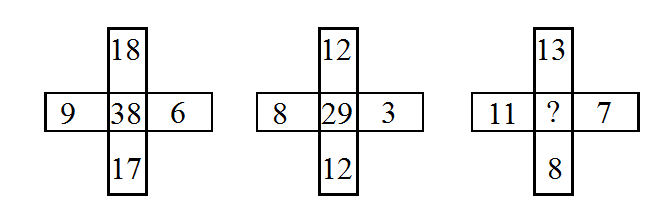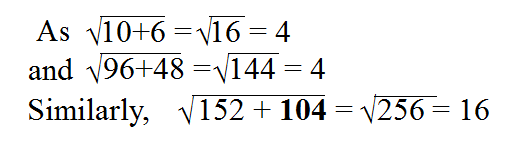# Number Puzzles - Questions with Answers for SSC Exam | Page-4

Questions

### Find the missing term in each of the following:

22A 250
B 930
C 390
D 640

Solution:

We have (12 + 42 + 52 + 32) × 10 = 510

(32 + 42 + 62 + 22) × 10 = 650

Hence, missing term = (42 + 22 + 82 + 32) × 10 = 930

23A 17
B 19
C 18
D 11

Solution:

As 36, 12 is divisible by 4

and 32, 16 is divisible by 8

Similarly, 17, 17 is divisible by 17

24A 230
B 125
C 135
D 209

Solution:

We have 82 = 64 and 83 = 512

42 = 16 and 43 = 64

Hence, missing number = 52 = 25 and 53 = 125

25A 35
B 30
C 25
D 20

Solution:

As (18 + 17) + (9 - 6) = 38

and (12 + 12) + (8 - 3) = 29

So, the missing term = (13 + 8) + (11 - 7) = 25

26A 101
B 102
C 103
D 104

Solution:27A 3
B 5
C 8
D 7

Solution:

As, 9 × 7 × 5 = 315

and 5 × 3 × 8 = 120

Similarly, 8 × 7 × ? = 168 ⇒ 168 ÷ 56 = 3

28A 55
B 68
C 79
D 86

Solution:

As, (35 + 20 + 28) - 43 = 40

and (28 + 11 + 16) - 35 = 40

So the missing number = (25 + 35 + 40) - 32 = 68